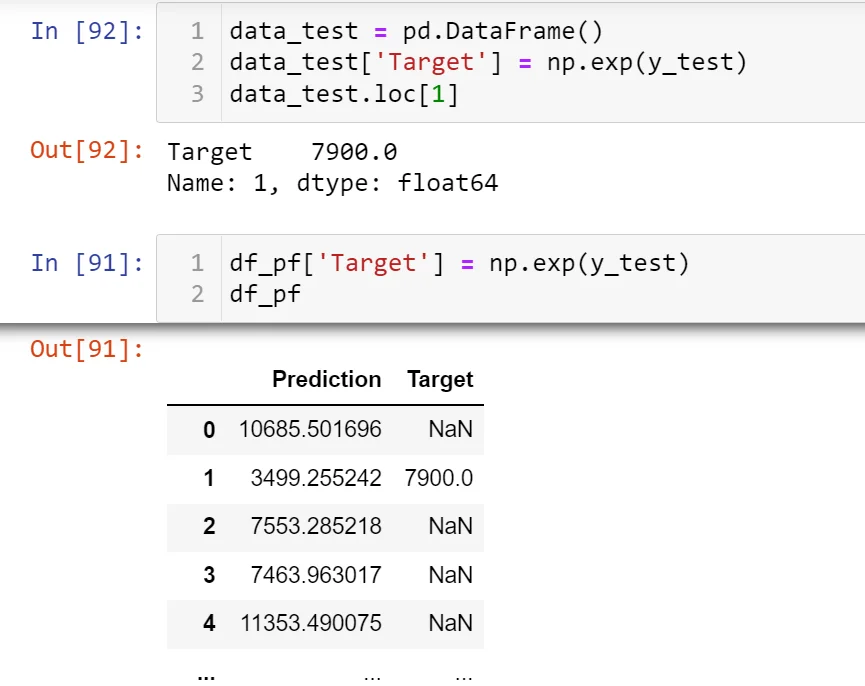Super learner

26 Sept 2022

Posted on:

23 Sept 2022

0

# Resolved:Cannot understand why resetting indexes makes NaNs disappear

Can you better explain why initially np.exp(y_test) gives NaN values and resetting the indexes solves this issue? Thanks

Instructor
Posted on:

26 Sept 2022

1

Hey Alessandro,

Thank you for reaching out!

Let's try to understand what each of the variables stores.

The variable `targets` stores the target values, with each of these values corresponding to a unique index. In the `targets` variable, the indices are arranged in an ascending order.

The `train_test_split` method reserves some of the observations for training and some - for testing. It does that by shuffling the samples, but preserving their original indices.

Now, `y_hat_test` is an array of numbers representing the predictions from `x_test`. Most importantly, `y_hat_test` doesn't know anything about the indexing of `x_test`. Therefore, once we add `np.exp(y_hat_test)` as a column of a `DataFrame`, the indexing naturally starts from 0 and goes down to 773 in an ascending fashion.

Now, imagine what happens when we add `np.exp(y_test)` as a second column to the same `DataFrame` object. `pandas` will try to match the indices of `y_hat_test` (ranging from 0 to 773) to those of `y_test` (randomly drawn from the `targets` variable). However, `y_test` contains indices that are larger than 773. Additionally, some indices between 0 and 773 will not be included. Let's show that this is indeed the case.

In the code below, right after the definition of the `df_pf` `DataFrame`, I have created another one called `data_test` that stores only the (exponential of the) values of `y_test` together with their original indices.From the output of the `df_pf` `DataFrame`, we can see that index 1 gives a non-null value in the Target column, while index 2 corresponds to null target. By typing

``````data_test.loc
``````

we see that the output is indeed 7900.0, as in the `DataFrame` below. Typing

``````data_test.loc
``````

on the other hand, returns in an error. The reason is that there is no value in `data_test` with an index of 2.

To resolve this issue, we reset the indices of the `y_test` variable, such that they start from 0 and go down to 773 in an ascending order. In that way, each prediction will have a corresponding target with the same index.

Hope this helps! Let me know if anything remains unclear.

Kind regards,
365 Hristina

Super learner
Posted on:

26 Sept 2022

0

I have to play a bit around with it, but I think it's quite clear. Thank you!